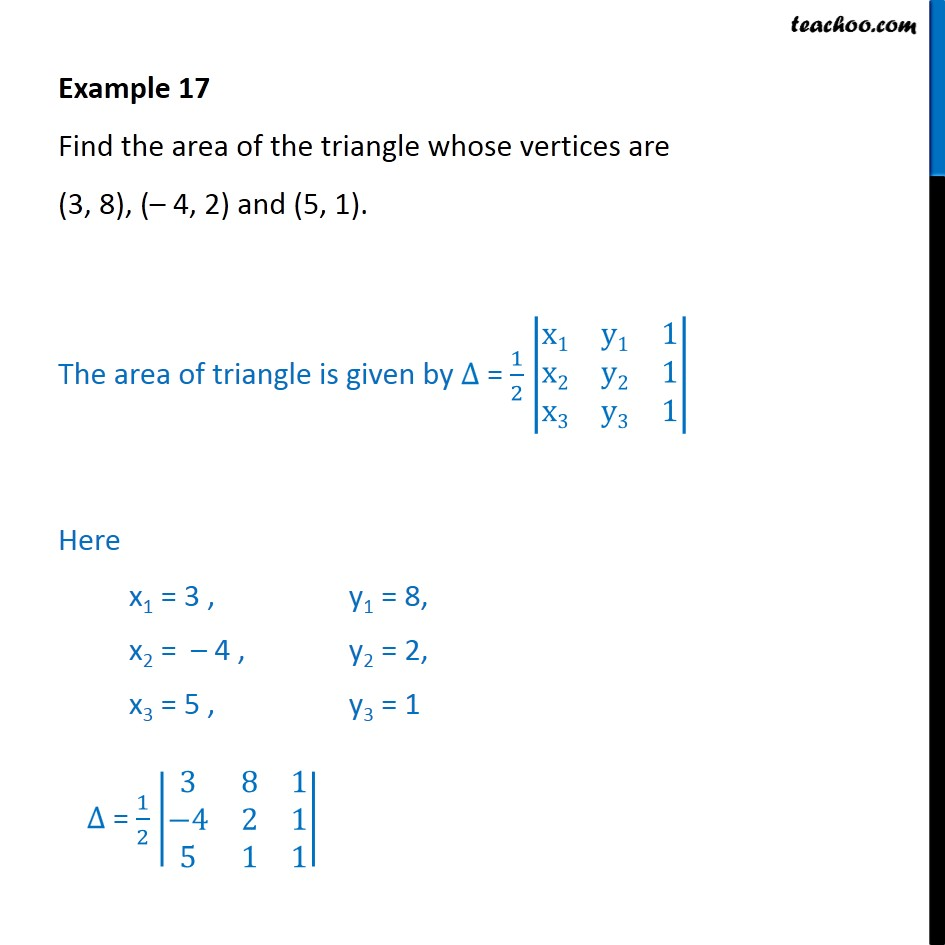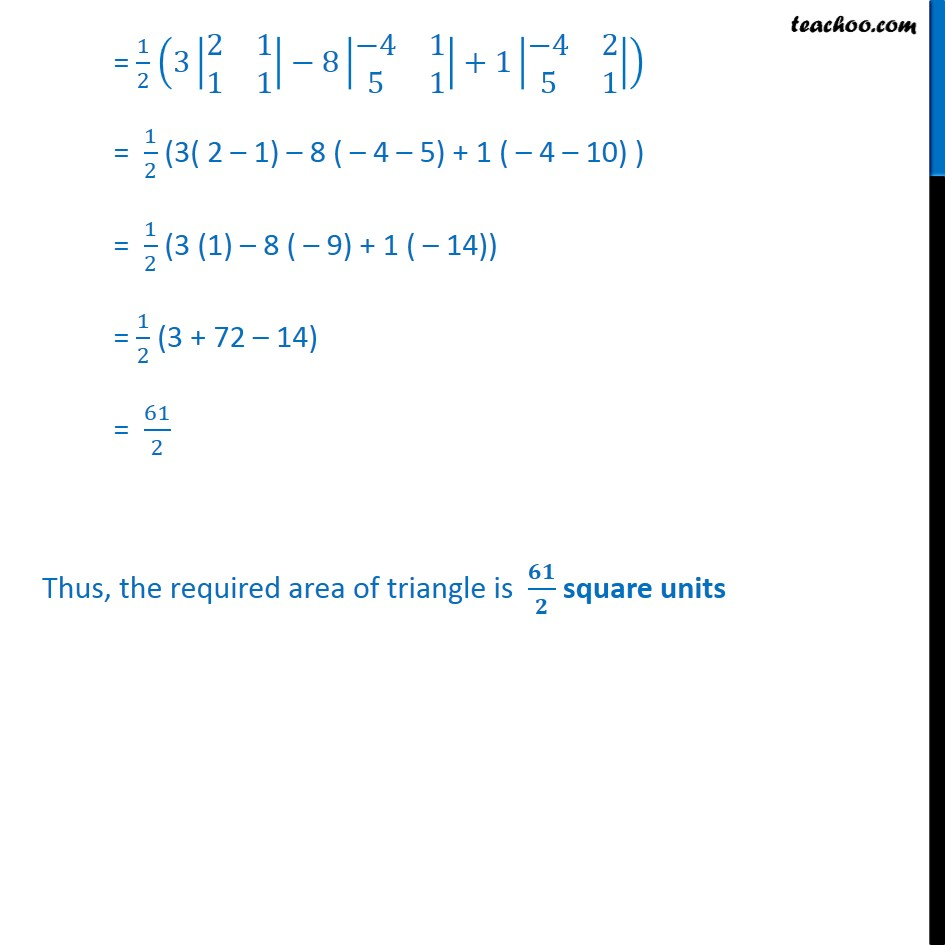Examples

Chapter 4 Class 12 Determinants
Serial order wiseGet live Maths 1-on-1 Classs - Class 6 to 12

### Transcript

Example 17 Find the area of the triangle whose vertices are (3, 8), ( 4, 2) and (5, 1). The area of triangle is given by = 1 2 x1 y1 1 x2 y2 1 x3 y3 1 Here x1 = 3 , y1 = 8, x2 = 4 , y2 = 2, x3 = 5 , y3 = 1 = 1 2 3 8 1 4 2 1 5 1 1 = 1 2 3 2 1 1 1 8 4 1 5 1 +1 4 2 5 1 = 1 2 (3( 2 1) 8 ( 4 5) + 1 ( 4 10) ) = 1 2 (3 (1) 8 ( 9) + 1 ( 14)) = 1 2 (3 + 72 14) = 61 2 Thus, the required area of triangle is square units